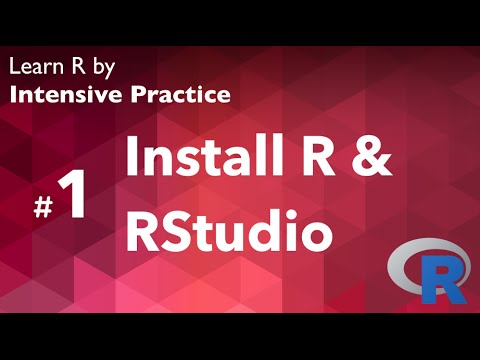#### data vizLearn R

R is a popular language used by data scientists and researchers. If you are working with data, R is a fantastic language to learn.

RAdvanced Data Science with IBM from IBM. As a coursera certified specialization completer you will have a proven deep understanding on massive parallel data processing, data exploration and visualization, and advanced machine learning.

RIntroduction to R for Data Science

Learn the R statistical programming language, the lingua franca of data science in this hands-on course.

RR, ggplot, and Simple Linear Regression

Begin to use R and ggplot while learning the basics of linear regression

RR Tutorial 01: Installing R and RStudio

Learn R programming language for beginner level.

RProgramming with R for Data Science

Programming with R for Data Science

RMaps with R Leaflet

Transform your data into interactive maps! - Free Course

RData Science

Data Science from Johns Hopkins University. Ask the right questions, manipulate data sets, and create visualizations to communicate results. This Specialization covers the concepts and tools you'll need throughout the entire data science

RIntroduction to R for Data Science

Introduction to R for Data Science

RPolynomial Regression, R, and ggplot

Learn how to write and graph functions in R and how to fit polynomials to data sets.

R